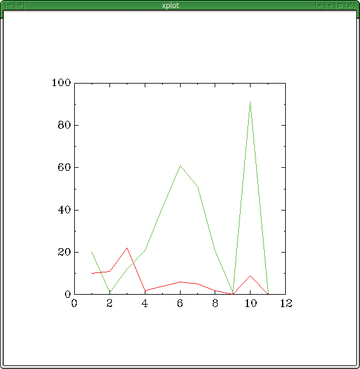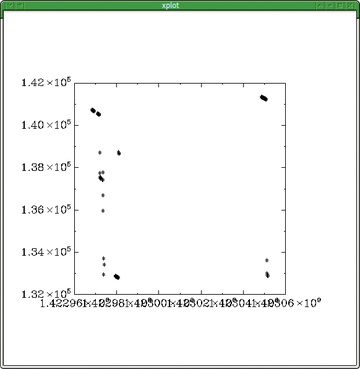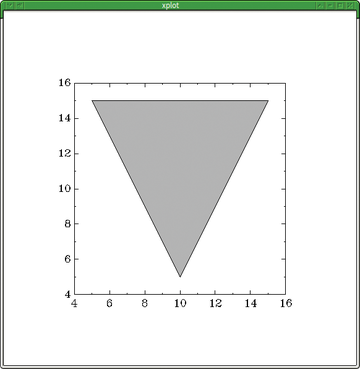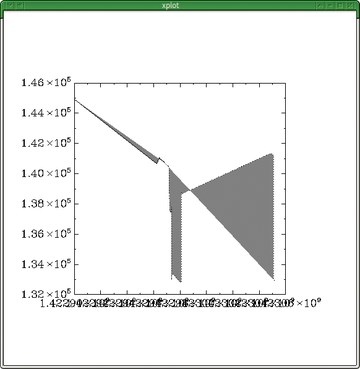# Creating informative graphs with plotutils

#### Curves and Lines

If empty lines appear in the input, Graph interprets them as the start of a new plot and displays the plot correspondingly (Figure 2). The program behaves similarly when data is derived from multiple sources (as shown in the first line of the following example). To distinguish the two plots, give the second one a different color using the -C option as shown in the second line below:

```\$ graph -TX /tmp/tst /tmp/tst1
\$ graph -TX -C /tmp/tst2 /tmp/tst3```

See Figures 3 and 4 for examples.Figure 2: Multiple plot curves are distinguished by different line types, even when they don't overlap.Figure 3: The -C option adds color to plot curves.Figure 4: The -S marks plots with data point symbols, even if the plot line is hidden (-m0).

#### Axis and Surfaces

The -a option automatically labels the X axis and can process data with just Y-axis values. This helps when there are constant values or only one coordinate indicated. An example is described in the "Problems with Date Representation" box. The -a option increments the X value for each given Y value. You can add arguments or (if values are missing) let Graph calculate it for you. The two arguments you can set for -a are the first one for the X-axis increment and the second for its lower limit value, as in the example … -a 100 10 … .

Problems with Date Representation

If you're working with time-dependent data, you have two possibilities. The first is to use absolute time with the format string +"%s" , which indicates the number of seconds since the beginning of the Unix epoch, January 1, 1970. The downside is that these values have become quite high over the years. The current date is thereby measured in terms of about 1.4 million seconds when using +"%s" , thus needing to be converted. In scripts, you would use Bc or Dc, in case the shell syntax isn't enough.

The second option is to use other date-formatting strings, such as the %d day number or the %I hour. For this approach, you need to ensure that these values aren't repeated (i.e., you need to delete the input files for Graph often). Alternatively, you can go back to using the -a option in Graph that creates the automatic X-axis values, as long as the distance between values remain the same.

Also interesting is the -q option, whereby Graph fills the polygon formed by the plot line segments with varying shading – with 0.5 halfway between solid and white, as in the following command that generates the plot shown in Figure 5.

`\$ echo 10 5  5 15  15 15  10 5 | graph -TX -q 0.5`Figure 5: The -q option creates variously shaded surfaces in the plots.

To create a closed surface, the starting and ending plot values have to be identical. The -q argument sets the density of the fill shading, with higher values creating darker shading. Using the -C adds color to the shading. A bit more complicated is filling open curves using -q . In this case, Graph adds a virtual line from the starting and ending points of the curve as the base for the filled surface (Figure 6).Figure 6: The fill function applies to curves as well, which are closed automatically with a virtual line from start to end point.

Express-Checkout as PDF

Pages: 5

Price \$0.99
(incl. VAT)